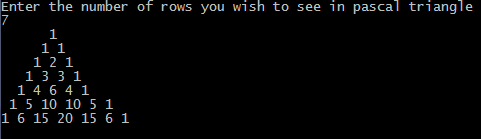## Print this Job Post# C program to print Pascal triangle

Pascal Triangle in c: C program to print Pascal triangle which you might have studied in Binomial Theorem in Mathematics. Number of rows of Pascal triangle to print is entered by the user. First four rows of Pascal triangle are shown below :-
1
11
121
1331

Pascal triangle in c
#include <stdio.h>
#include<conio.h>
long factorial(int);
void main()
{
int i, n, c;
clrscr();
printf("Enter the number of rows you wish to see in pascal triangle\n");
scanf("%d",&n);
for ( i = 0 ; i < n ; i++ )
{
for ( c = 0 ; c <= ( n - i - 2 ) ; c++ )
printf(" ");
for( c = 0 ; c <= i ; c++ )
printf("%ld ",factorial(i)/(factorial(c)*factorial(i-c)));
printf("\n");
}
getch();
}
long factorial(int n)
{
int c;
long result = 1;
for( c = 1 ; c <= n ; c++ )
result = result*c;
return ( result );
}

## Output of program: